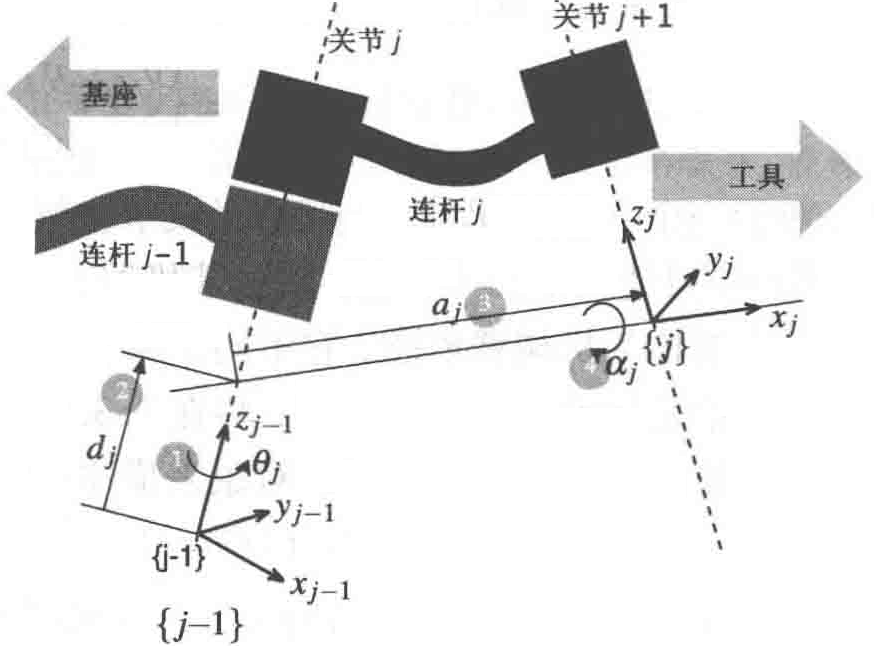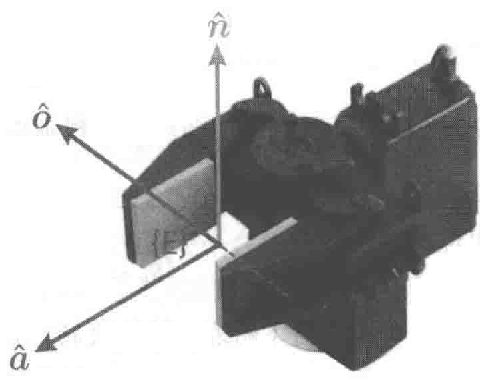$N$ 个关节（编号从 $1$  $N$）的机械臂具有 $N+1$ 个连杆（编号从 $0$  $N$）。连杆 $0$ 是机械臂的基座，连杆 $N$ 则固接末端执行器或者工具。关节 $j$ 将连杆 $j-1$ 连接到连杆上，因此关节 $j$ 带动连杆 \$j。连杆被看作一个刚体，它确定了其相邻两个关节轴线的空间关系。一个连杆由长度 $a_j$和扭转角 $\alpha_j$，两个参数来描述。同样，一个关节也由两个参数来描述，其中连杆偏移 $d_j$ 是指沿着关节轴线从一个连杆坐标系到另一个连杆坐标系的距离，而关节角 $\theta_j$，则是指一个连杆相对于另一个连杆关于关节轴线的转动角度。$^{j-1}A_j(\theta_j,d_j,a_j,\alpha_j)=T_{Rz}(\theta_j)T_z(d_j)T_x(a_j)T_{Rx}(\alpha_j)$展开如下
$^{j-1}A_j=\left( \begin{matrix} \cos \theta _j& -\sin \theta _j\cos \alpha _j& \sin \theta _j\sin \alpha _j& a_j\cos \theta _j\\ \sin \theta _j& \cos \theta _j\cos \alpha _j& -\cos \theta _j\sin \alpha _j& a_j\sin \theta _j\\ 0& \sin \alpha _j& \cos \alpha _j& d_j\\ 0& 0& 0& 1\\ \end{matrix} \right)$

$q_j=\left\{ \begin{array}{l} \theta _j,\quad\sigma_j=0转动关节\\[1em] d_j,\quad\sigma_j=1移动关节\\ \end{array} \right.$对于一个 $N$ 轴的机器人，其广义关节坐标为 $q\in\mathcal{C}$，其中 $\mathcal{C}\subset\mathbb{R}^N$ 被称为关节空间或者位形空间。一般情况下，一个全旋转关节机器人 $\mathcal{C}\subset\mathbb{S}^N$ 的关节坐标被称为关节角度。这种关节坐标同时也被称为机械臂姿态，它不同于属于笛卡儿姿态 $\xi\in SE(3)$ 的末端执行器的姿态。


>> L = Link([0, 0.1, 0.2, pi/2, 0])
L =
theta=q, d=0.1, a=0.2, alpha=1.571, offset=0 (R,stdDH)


Link对象的显示值不仅给出了其运动学参数，还表示了其他的一些状态，例如它是一个转动关节（标签“R”），以及使用了标准的 D-H 参数表达（标签“stdDH”）。

$q=0.5$ 时，连杆变换矩阵如下：

>> L.A(0.5)
ans =
0.8776   -0.0000    0.4794    0.1755
0.4794    0.0000   -0.8776    0.0959
0    1.0000    0.0000    0.1000
0         0         0    1.0000


>> L.RP
ans =
'R'


>> L.a
ans =
0.2000


>> L.offset = 0.5;
>> L.A(0)
ans =
0.8776   -0.0000    0.4794    0.1755
0.4794    0.0000   -0.8776    0.0959
0    1.0000    0.0000    0.1000
0         0         0    1.0000